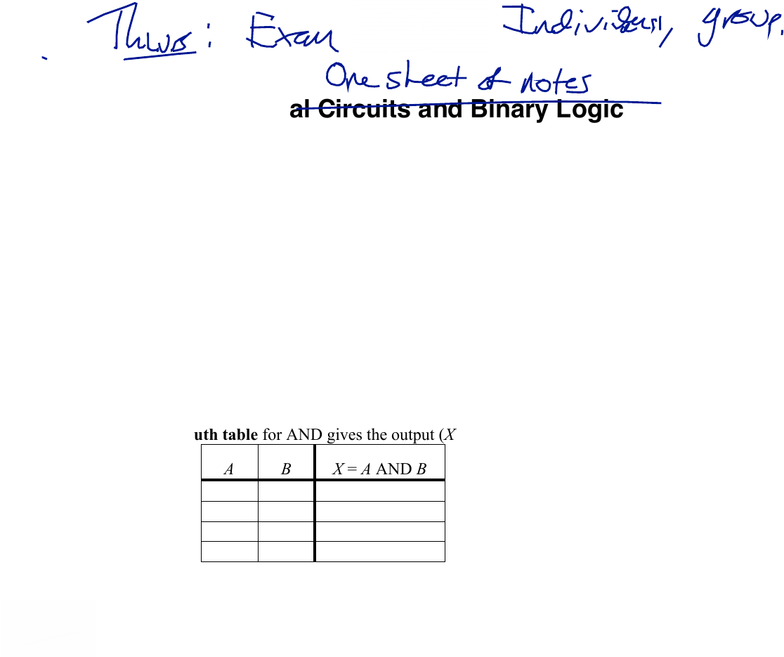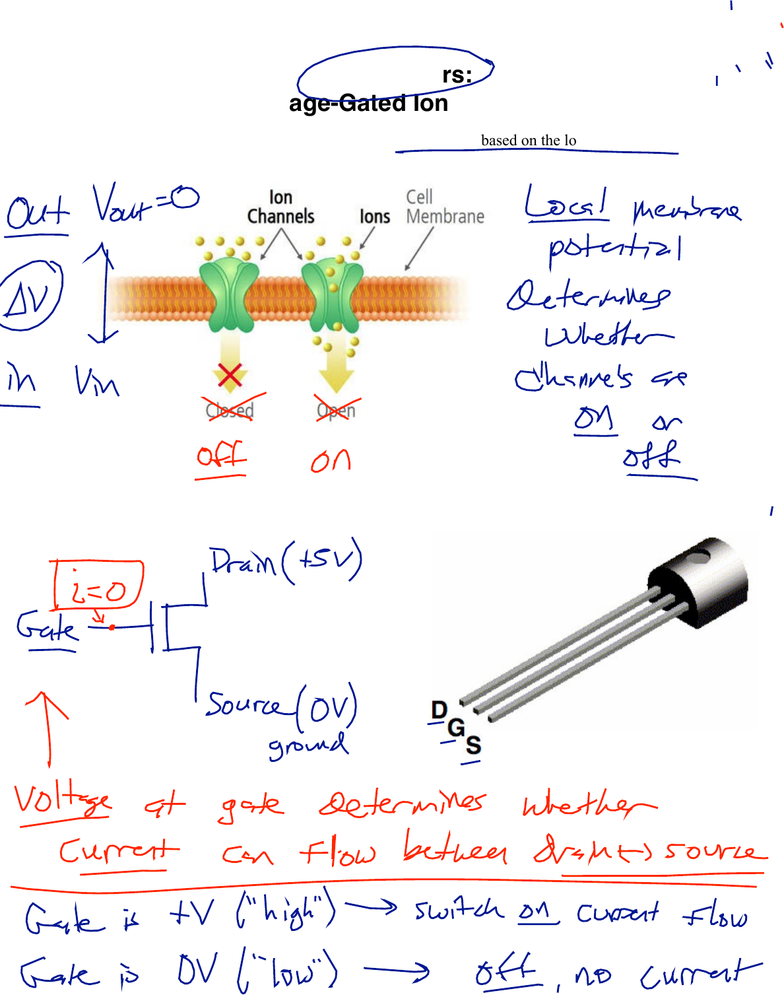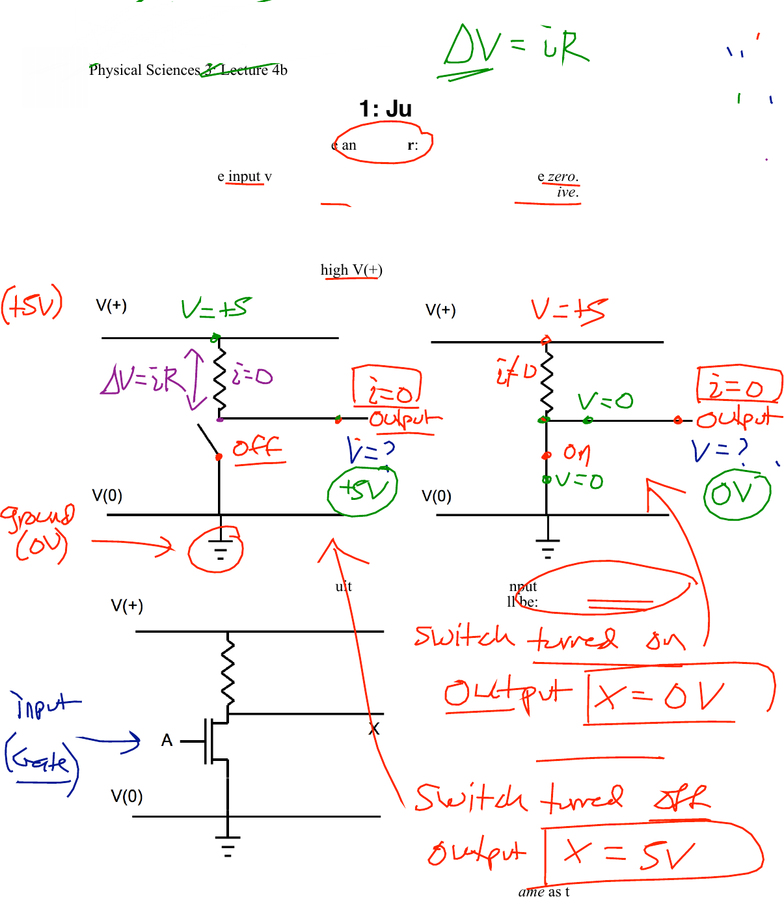Study Guides (400,000)
US (230,000)
Harvard (700)
(20)
Final

# Physical Sciences 3 Lecture 9: Lecture 4bExam

Department
Physical Sciences
Course Code
Physical Sciences 3
Professor
Roxanne Guenette
Study Guide
Final

This preview shows pages 1-3. to view the full 10 pages of the document.Physical Sciences 3: Lecture 4b
February 26, 2019
1
Digital Circuits and Binary Logic
A transistor is an electronic device that can act as a switch. Every transistor has three
terminals: source, drain, and gate. The voltage at the gate controls the flow of current
between the source and drain.
Gate is high (+V); switch is on; current can flow between source and drain
Gate is low (zero); switch is off; current cannot flow between source and drain
We can use the binary number system (0 and 1) to represent numbers for arithmetic, or
to represent logical statements (false = 0; true = 1). We can operate on logical statements
using operations like AND, OR, or NOT, where these operations are defined using “truth
tables.”
For instance, the logical operation AND takes two inputs (A and B), and provides an
output (X) according to the following rules:
X is true (1) if both A and B are true (1), and will be false (0) otherwise.
The truth table for AND gives the output (X) for all possible combinations of A and B:
A
B
X = A AND B
0
0
0
0
1
0
1
0
0
1
1
1
We can use transistors and resistors to construct actual circuits that implement any logic
gate. The basic building blocks are the circuits for NOT, NAND, and NOR.
Learning objectives: After this lecture, you will be able to…
1. Describe the operation of a transistor: how it acts like a “voltage-gated” switch. Explain
the role of the three terminals (source, drain, and gate).
2. Design a circuit using transistors and resistors that acts as an inverter (NOT). Explain
how it works.
3. Convert decimal numbers into binary (and vice versa); add binary numbers.
4. Use binary digits (“bits”) to represent logical statements (0 = false, 1 = true), and
combine these logical statements using AND, OR, NOT, and other logical operations.
5. Write truth tables for various logical operations, and combinations of logical operations.
6. Draw and interpret logic gate diagrams that show combinations of logical operations.
7. Design a logic circuit (the “half-adder”) that can add two one-digit binary numbers.
8. Design circuits using transistors and resistors to implement various logical operations.
9. Write truth tables for logic gate diagrams, or for transistor circuit diagrams.

Only pages 1-3 are available for preview. Some parts have been intentionally blurred.Physical Sciences 3: Lecture 4b
February 26, 2019
2
Transistors:
“Voltage-Gated Ion Channels”
In a neuron, there are ion channels that open or close based on the local membrane
potential. What do we mean by this?
An electronic device known as a transistor can act like a voltage-gated channel. What is
a transistor? How does it appear in a circuit diagram?

Only pages 1-3 are available for preview. Some parts have been intentionally blurred.Physical Sciences 3: Lecture 4b
February 26, 2019
3
Activity 1: Just Say No
We can use a transistor to create an inverter:
- if the input voltage is positive, the output voltage will be zero.
- if the input voltage is zero, the output voltage will be positive.
1. In all the logic circuits we will consider, the output (the middle wire at right) draws no
current. This is extremely important. Using that fact, along with Ohm’s Law and your
understanding of circuits, determine the potential at the output in each of the following
cases. (Hint: it will either be high V(+), or it will be zero…)
2. Now consider the transistor circuit below. If the transistor input (A) is high (positive),
the output (X) will be:
If the transistor input (A) is low (zero), the
output (X) will be:
Bonus! Design a circuit with two transistors where the output is the same as the input.